# # 图解原型链及其继承优缺点

## # 引言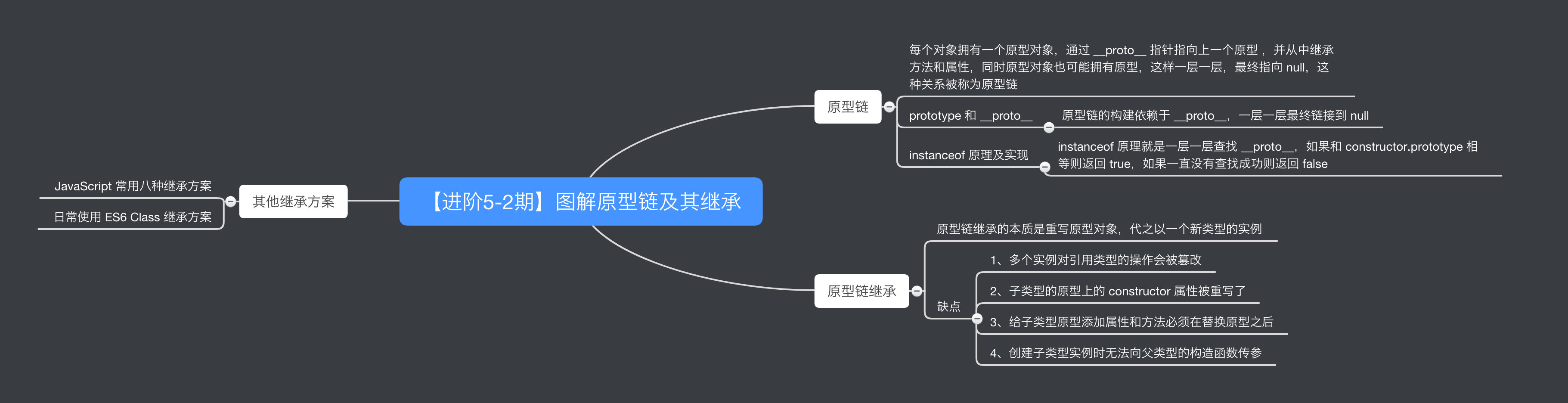## # 原型链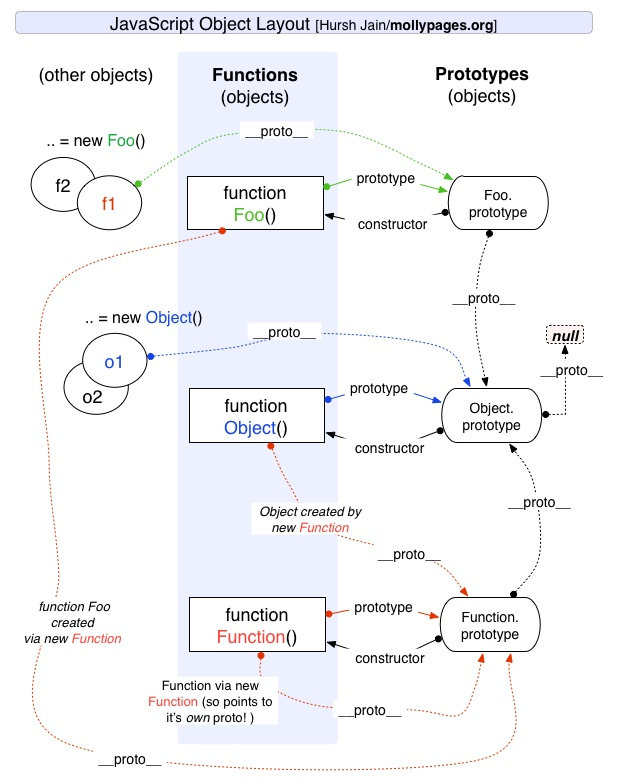``````// 木易杨
function Foo(name) {
this.name = name;
}
Foo.prototype.getName = function() {
return this.name;
}
Foo.prototype.length = 3;
let foo = new Foo('muyiy'); // 相当于 foo.__proto__ = Foo.prototype
console.dir(foo);
``````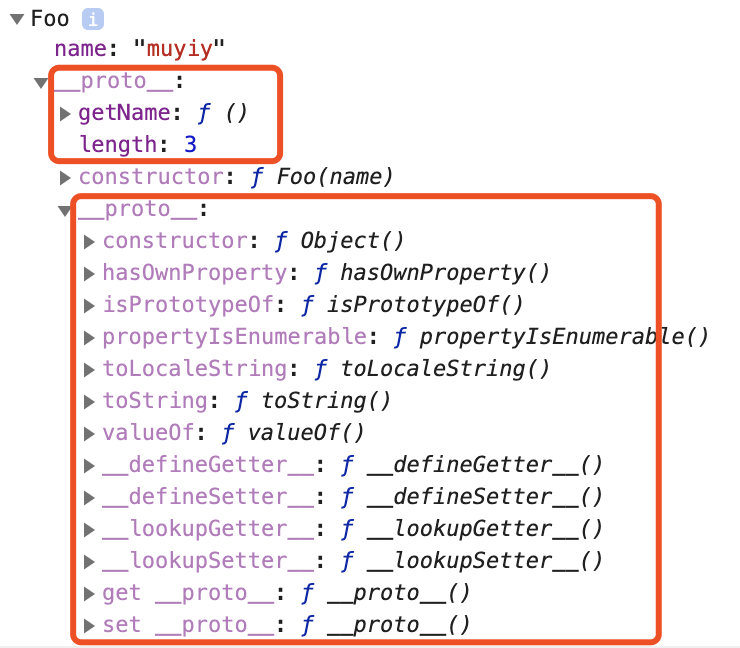• 首先检查 `foo` 对象是否具有可用的 `valueOf()` 方法。
• 如果没有，则检查 `foo` 对象的原型对象（即 `Foo.prototype`）是否具有可用的 `valueof()` 方法。
• 如果没有，则检查 `Foo.prototype` 所指向的对象的原型对象（即 `Object.prototype`）是否具有可用的 `valueOf()` 方法。这里有这个方法，于是该方法被调用。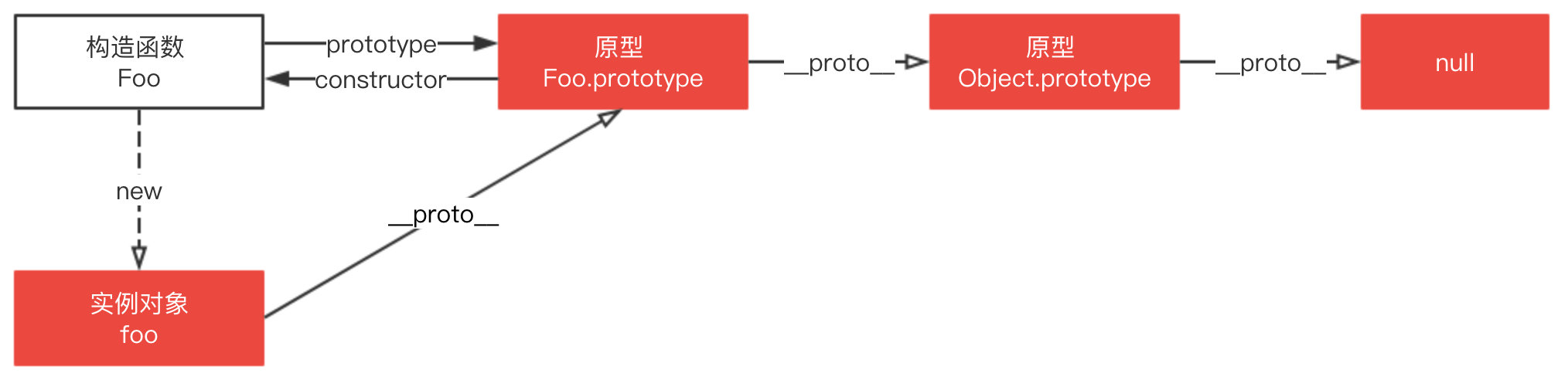### #`prototype` 和 `__proto__`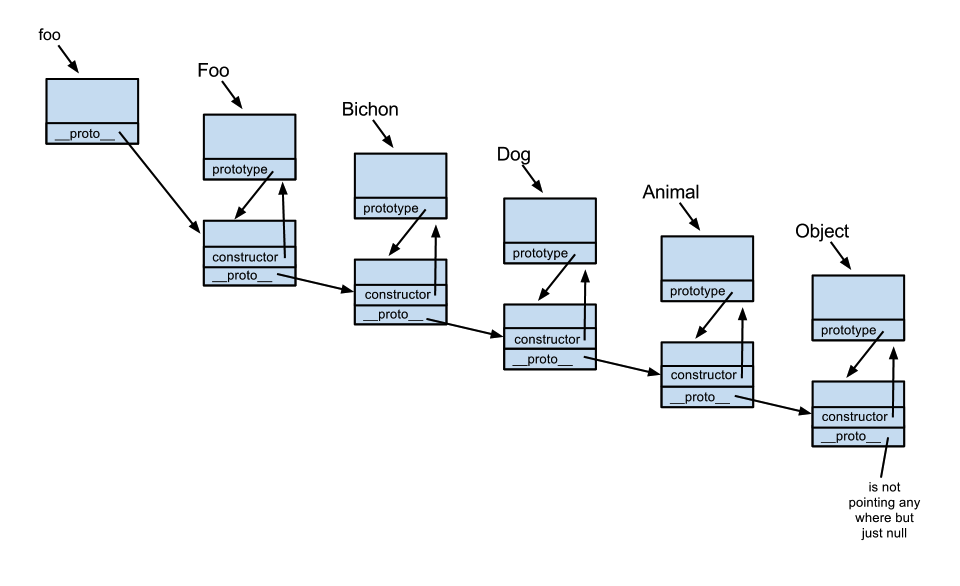.png)

https://kenneth-kin-lum.blogspot.com/2012/10/javascripts-pseudo-classical.html

`Foo.prototype` 中的 `prototype` 并没有构建成一条原型链，其只是指向原型链中的某一处。原型链的构建依赖于 `__proto__`，如上图通过 `foo.__proto__` 指向 `Foo.prototype``foo.__proto__.__proto__` 指向 `Bichon.prototype`，如此一层一层最终链接到 `null`

``````// 木易杨
function Foo() {
return 'foo';
}
Foo.prototype.method = function() {
return 'method';
}
function Bar() {
return 'bar';
}
Bar.prototype = Foo; // Bar.prototype 指向到函数
let bar = new Bar();
console.dir(bar);

bar.method(); // Uncaught TypeError: bar.method is not a function
``````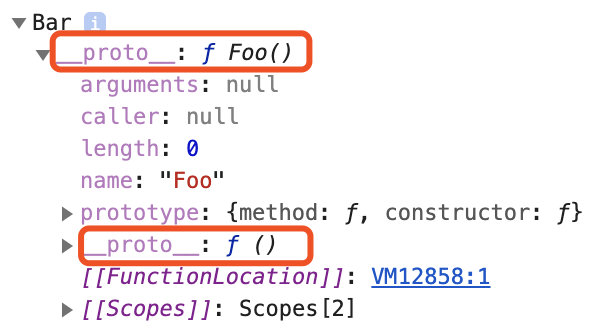### # instanceof 原理及实现

`instanceof` 运算符用来检测 `constructor.prototype` 是否存在于参数 `object` 的原型链上。

``````// 木易杨
function C(){}
function D(){}

var o = new C();

o instanceof C; // true，因为 Object.getPrototypeOf(o) === C.prototype
o instanceof D; // false，因为 D.prototype 不在 o 的原型链上
``````

instanceof 原理就是一层一层查找 `__proto__`，如果和 `constructor.prototype` 相等则返回 true，如果一直没有查找成功则返回 false。

``````instance.[__proto__...] === instance.constructor.prototype
``````

``````// 木易杨
function instance_of(L, R) {//L 表示左表达式，R 表示右表达式
var O = R.prototype;// 取 R 的显示原型
L = L.__proto__;// 取 L 的隐式原型
while (true) {
// Object.prototype.__proto__ === null
if (L === null)
return false;
if (O === L)// 这里重点：当 O 严格等于 L 时，返回 true
return true;
L = L.__proto__;
}
}

// 测试
function C(){}
function D(){}

var o = new C();

instance_of(o, C); // true
instance_of(o, D); // false
``````

## # 原型链继承

``````// 木易杨
function Animal() {
this.value = 'animal';
}

Animal.prototype.run = function() {
return this.value + ' is runing';
}

function Cat() {}

// 这里是关键，创建 Animal 的实例，并将该实例赋值给 Cat.prototype
// 相当于 Cat.prototype.__proto__ = Animal.prototype
Cat.prototype = new Animal();

var instance = new Cat();
instance.value = 'cat'; // 创建 instance 的自身属性 value
console.log(instance.run()); // cat is runing
``````

• 1、多个实例对引用类型的操作会被篡改
• 2、子类型的原型上的 constructor 属性被重写了
• 3、给子类型原型添加属性和方法必须在替换原型之后
• 4、创建子类型实例时无法向父类型的构造函数传参

### # 问题 1

``````// 木易杨
function Animal(){
this.names = ["cat", "dog"];
}
function Cat(){}

Cat.prototype = new Animal();

var instance1 = new Cat();
instance1.names.push("tiger");
console.log(instance1.names); // ["cat", "dog", "tiger"]

var instance2 = new Cat();
console.log(instance2.names); // ["cat", "dog", "tiger"]
``````

### # 问题 2

``````Cat.prototype = new Animal();
Cat.prototype.constructor === Animal
// true
``````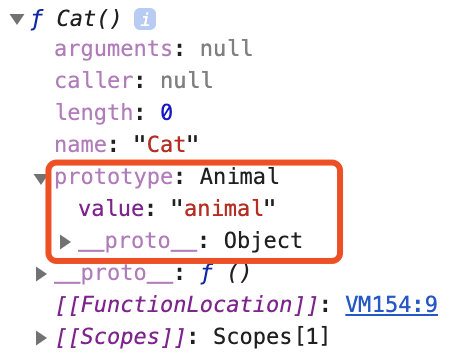``````// 木易杨
function Animal() {
this.value = 'animal';
}

Animal.prototype.run = function() {
return this.value + ' is runing';
}

function Cat() {}
Cat.prototype = new Animal();

// 新增，重写 Cat.prototype 的 constructor 属性，指向自己的构造函数 Cat
Cat.prototype.constructor = Cat;
``````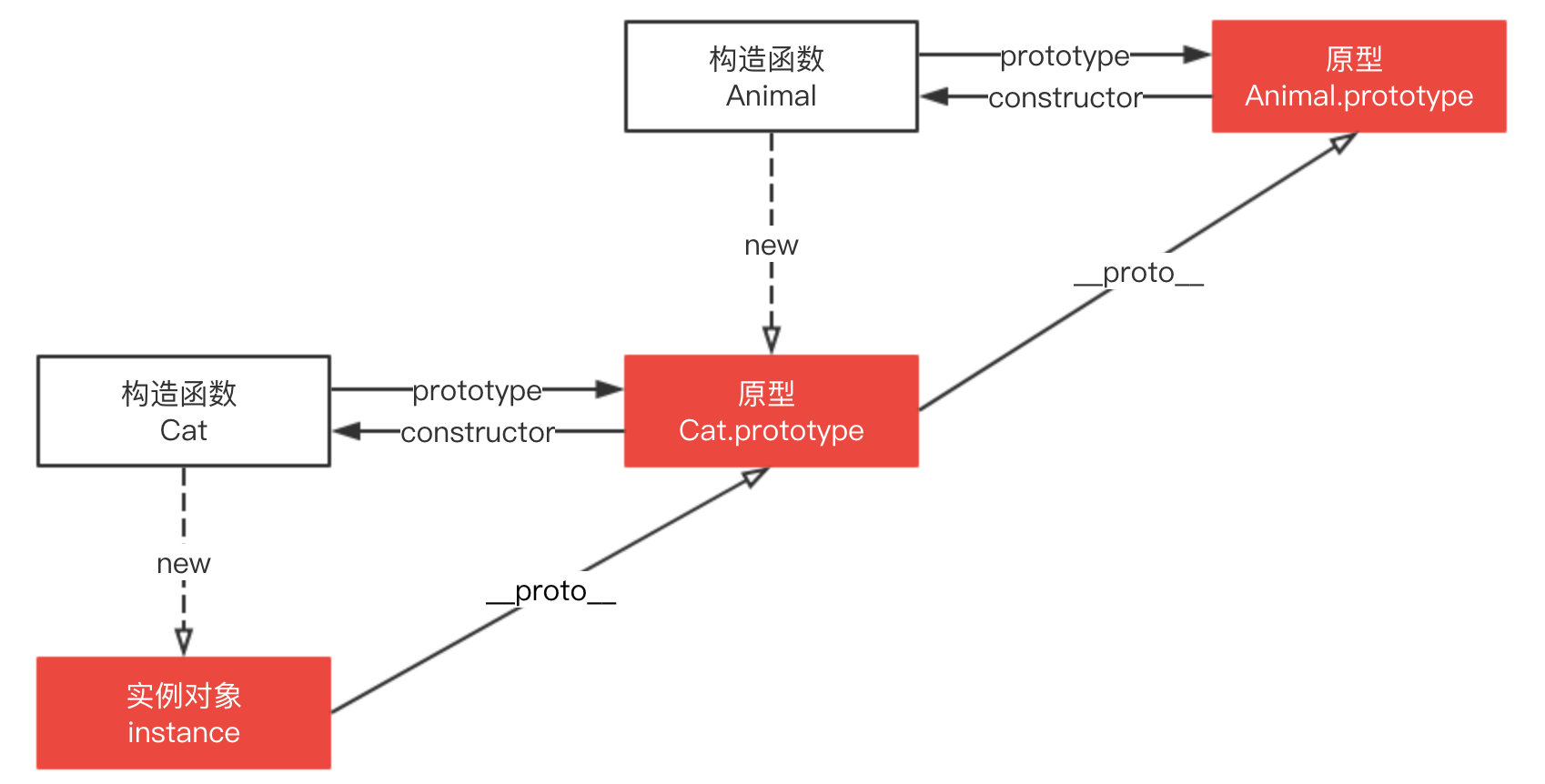### # 问题 3

``````// 木易杨
function Animal() {
this.value = 'animal';
}

Animal.prototype.run = function() {
return this.value + ' is runing';
}

function Cat() {}
Cat.prototype = new Animal();
Cat.prototype.constructor = Cat;

// 新增
Cat.prototype.getValue = function() {
return this.value;
}

var instance = new Cat();
instance.value = 'cat';
console.log(instance.getValue()); // cat
``````

### # 属性遮蔽

``````// 木易杨
function Animal() {
this.value = 'animal';
}

Animal.prototype.run = function() {
return this.value + ' is runing';
}

function Cat() {}
Cat.prototype = new Animal();
Cat.prototype.constructor = Cat;

// 新增
Cat.prototype.run = function() {
return 'cat cat cat';
}

var instance = new Cat();
instance.value = 'cat';
console.log(instance.run()); // cat cat cat
``````

``````// 接上
console.log(instance.__proto__.__proto__.run()); // undefined is runing
``````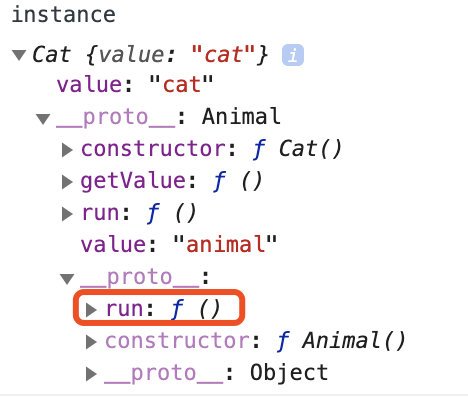## # 扩展题

Object.prototype.toString.call() 、 instanceof 以及 Array.isArray()

## # 小结

• 每个对象拥有一个原型对象，通过 `__proto__` 指针指向上一个原型 ，并从中继承方法和属性，同时原型对象也可能拥有原型，这样一层一层，最终指向 `null`，这种关系被称为**原型链 **
• 当访问一个对象的属性 / 方法时，它不仅仅在该对象上查找，还会查找该对象的原型，以及该对象的原型的原型，一层一层向上查找，直到找到一个名字匹配的属性 / 方法或到达原型链的末尾（`null`）。
• 原型链的构建依赖于 `__proto__`，一层一层最终链接到 `null`
• instanceof 原理就是一层一层查找 `__proto__`，如果和 `constructor.prototype` 相等则返回 true，如果一直没有查找成功则返回 false。
• 原型链继承的本质是重写原型对象，代之以一个新类型的实例

## # 参考

MDN 之对象原型

MDN 之继承与原型链

JavaScript Prototype Explained By Examples

JavaScript's Pseudo Classical Inheritance diagram